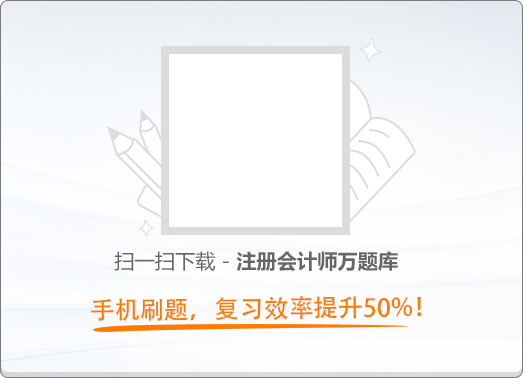150:002017年注册会计师《公司战略与风险管理》真题

1
(单项选择题)

• A.

家电业务的多数产品进入成熟期，公司在家电行业竞争优势显著，公司应加大投入力度，以维持优势地位

• B.

新能源行业发展潜力巨大，前景广阔，公司该领域竞争优势不足，公司应对新能源重点投资提高市场占有率

• C.

房地产进入寒冬期，公司房地产业务始终没有获利，应果断撤出

• D.

生物制药行业今年发展迅猛，公司收购的一家生物制药企业由弱到强，竞争优势迅猛展现，公司应在短期内优先供给其所需资源，支持发展

• A
• B
• C
• D

2
(单项选择题)

• A.

内部基准

• B.

过程或活动基准

• C.

一般基准

• D.

竞争性基准

• A
• B
• C
• D

3
(单项选择题)

• A.

该方法可能导致生产环节一旦出错则弥补空间小

• B.

该方法能够减少对供应商的依赖

• C.

该方法避免了因需求突然变动而导致大量产品无法出售的情况

• D.

该方法降低了存货变质、陈旧或过时的可能性

• A
• B
• C
• D

4
(单项选择题)

• A.

多元化战略

• B.

转向战略

• C.

放弃战略

• D.

产品开发战略

• A
• B
• C
• D

5
(单项选择题)

J国的S公司是一家全球500强企业，依靠严格的规章制度进行精细化管理，内部等级分明，决策权主要集中在上层，资历在员工晋升中发挥了重要作用。S公司的企业文化类型属于（　）。

• A.

任务导向型

• B.

人员导向型

• C.

角色导向型

• D.

权力导向型

• A
• B
• C
• D

6
(单项选择题)

• A.

差异化战略

• B.

成本领先战略

• C.

集中化战略

• D.

蓝海战略

• A
• B
• C
• D

7
(单项选择题)

2014年华阳矿业公司由于一次投资活动失败而遭受重大损失。后经相关机构调查发现，华阳公司投资失败的原因是该公司未建立重大投资项目按照规定的权限和程序实行集体决策或者联签的制度。按照内部控制缺陷的本质分类，华阳公司的上述缺陷属于（　）。

• A.

运行缺陷

• B.

其他缺陷

• C.

一般缺陷

• D.

设计缺陷

• A
• B
• C
• D

8
(单项选择题)

• A.

人口细分

• B.

心理细分

• C.

行为细分

• D.

地理细分

• A
• B
• C
• D

9
(单项选择题)

• A.

前向一体化战略

• B.

多元化战略

• C.

密集型战略

• D.

后向一体化战略

• A
• B
• C
• D

10
(单项选择题)

• A.

事业部制组织结构

• B.

战略业务单位组织结构

• C.

矩阵制组织结构

• D.

职能制组织结构

• A
• B
• C
• D

11
(单项选择题)

• A.

后推法

• B.

前推法

• C.

逆推法

• D.

正推法

• A
• B
• C
• D

12
(单项选择题)

• A.

损失融资

• B.

风险资本

• C.

保险

• D.

专业自保

• A
• B
• C
• D

13
(单项选择题)

• A.

建立商标信誉，开拓新销售渠道

• B.

保护现有市场，渗入别人的市场

• C.

选择区域市场，改善企业形象

• D.

广告宣传，开辟销售渠道

• A
• B
• C
• D

14
(单项选择题)

• A.

风险管理解决方案中的外部解决方案一般指外包

• B.

风险管理解决方案应有风险解决的具体目标和风险管理工具等方面的内容

• C.

落实风险管理解决方案必须认识到风险管理是企业价值创造的根本源泉

• D.

风险管理解决方案中的内部解决方案一般指风险管理策略

• A
• B
• C
• D

15
(单项选择题)

P公司是一家生产经营日化用品的跨国公司，其母公司设立在U国，在其他国家设立了20余个子公司。在该公司的经营过程中，母公司将产品的研发技术和新产品提供给各个子公司，子公司也会把在当地畅销的产品提供给母公司和其他子公司。P公司国际化经营的战略类型属于（　）。

• A.

跨国战略

• B.

全球化战略

• C.

多国本土化战略

• D.

国际战略

• A
• B
• C
• D

16
(单项选择题)

• A.

发展战略实施不到位

• B.

发展战略过于激进，脱离企业实际能力或偏离主业

• C.

发展战略因主观原因频繁变动

• D.

缺乏明确的发展战略

• A
• B
• C
• D

17
(单项选择题)

• A.

情景分析法

• B.

敏感性分析法

• C.

统计推论法

• D.

马尔科夫分析法

• A
• B
• C
• D

18
(单项选择题)

• A.

成长期

• B.

成熟期

• C.

衰退期

• D.

导入期

• A
• B
• C
• D

19
(单项选择题)

• A.

扭转型战略

• B.

多种经营战略

• C.

防御型战略

• D.

增长型战略

• A
• B
• C
• D

20
(单项选择题)

• A.

远期合约价格不能预先确定

• B.

期货价格不是通过公开竞价达成的

• C.

欧式期权只能在到期日执行

• D.

远期合约是标准化合约

• A
• B
• C
• D

21
(单项选择题)

• A.

技艺（知识）标准化

• B.

工作过程标准化

• C.

工作成果标准化

• D.

相互适应，自行调整

• A
• B
• C
• D

22
(单项选择题)

• A.

风险评估

• B.

控制活动

• C.

监控

• D.

控制环境

• A
• B
• C
• D

23
(单项选择题)

• A.

市场开发

• B.

相关多元化

• C.

市场渗透

• D.

产品开发

• A
• B
• C
• D

24
(单项选择题)

2010年，R国汽车制造商G公司预算随着绿色环保理念的普及和政府相关产业政策的退出，R公司的新能源汽车产业将迎来一个巨大的发展机遇；其本国竞争对手汽车制造商S公司，将凭借雄厚的资金实力和强大的科研能力，把投资和研发的重点转向新能源汽车领域。G公司对S公司的上述分析属于（　）。

• A.

成长能力分析

• B.

适应变化能力分析

• C.

快速反应能力分析

• D.

财务能力分析

• A
• B
• C
• D